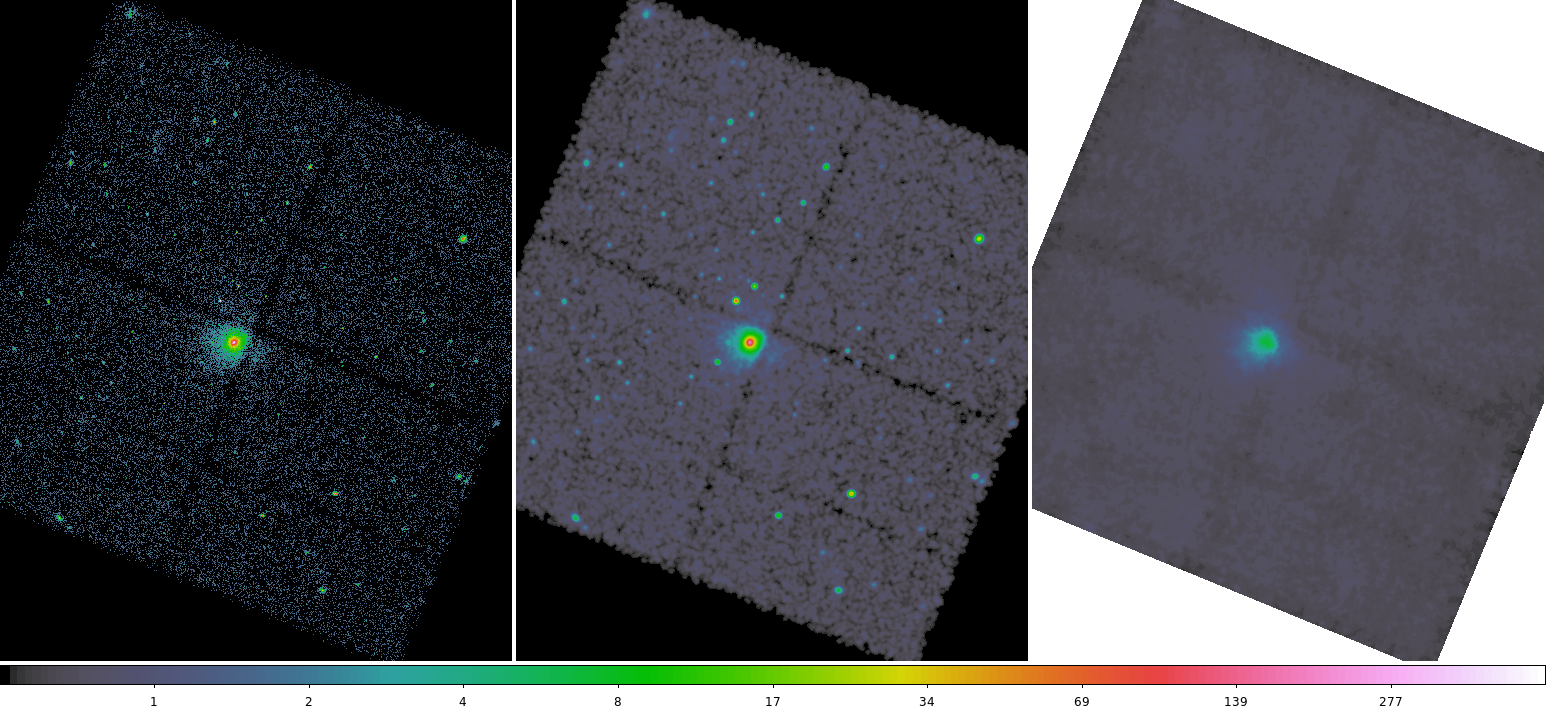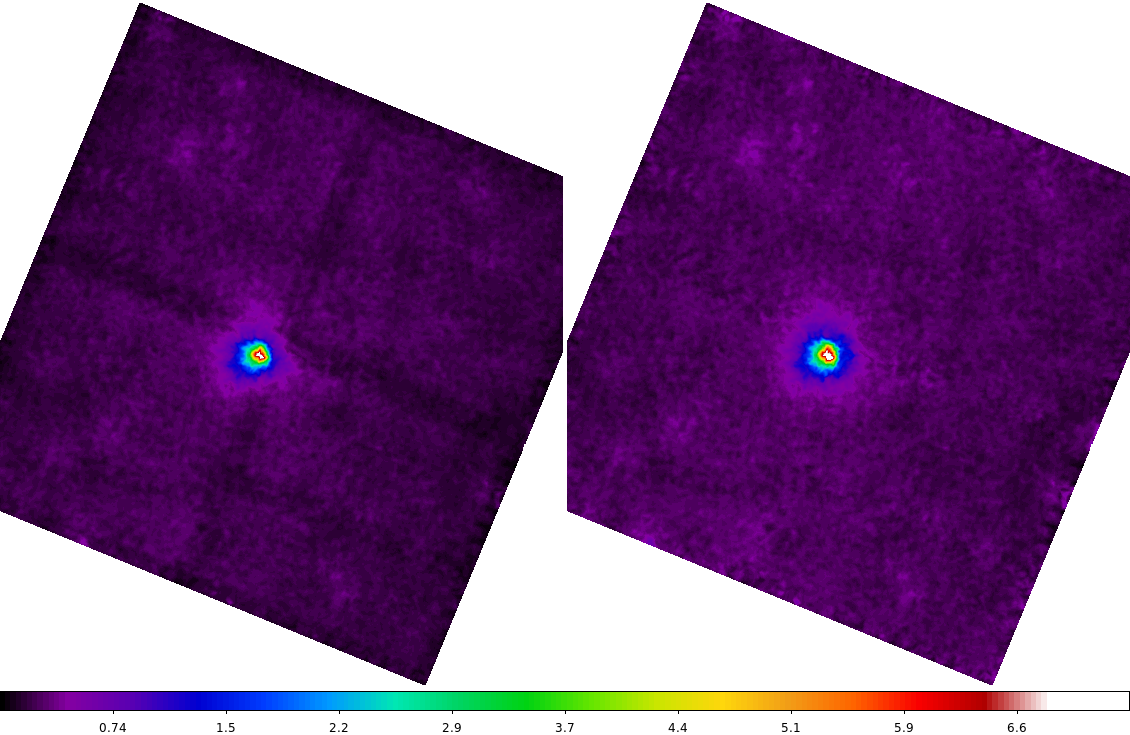# An Estimated Background Image## Overview

#### Synopsis:

This thread shows one method for making an estimated background image for a dataset. The resulting image can be used with any data analysis tool that takes a background image, e.g. wavdetect or dmextract.

you want to create a background image for an ACIS or HRC imaging observation. This method is suited to fields which contain a few point sources; it will not work well with very large extended objects.

Last Update: 14 Jan 2022 - Review for CIAO 4.14. Changed OBSID to 2254. Updated to use fluximage to create the image and exposure maps.

## Get Started

`unix% download_chandra_obsid 2254 `

## Make an Image of the Chip

```unix% fluximage acisf02254_repro_evt2.fits"[ccd_id=0:3]" 3C295 bin=2 band=broad clob+
Running fluximage
Version: 04 November 2021

Using CSC ACIS broad science energy band.

The output images will have 1367 by 1368 pixels, pixel size of 0.984 arcsec,
and cover x=2786.5:5520.5:2,y=2760.5:5496.5:2.

Running tasks in parallel with 4 processors.
Creating 4 aspect histograms for obsid 2254
Creating 4 instrument maps for obsid 2254
Creating 4 exposure maps for obsid 2254
Combining 4 exposure maps for obsid 2254
Thresholding data for obsid 2254
Exposure-correcting image for obsid 2254

The following files were created:

The clipped counts image is:

The observation FOV is:
3C295_2254.fov

The clipped exposure map is:

The exposure-corrected image is:
```

Next we filter the image with the field-of-view (FOV) file.

```unix% dmcopy "3C295_broad_thresh.img[sky=region(3C295_2254.fov)][opt full]" \
```

Filtering this way ensures that the edge of the detector is stored in the image and can be used by later processing tools.

## Convolve the Image

The counts image is first convolved with a 3 pixel sigma Gaussian by aconvolve to smooth out the zero-value pixels. This will produce better results from the median-value filter applied in the next step.

```unix% pset aconvolve infile=3C295_broad_dss.img
unix% pset aconvolve kernelspec="lib:gaus(2,5,1,3,3)"
unix% pset aconvolve meth=fft
unix% aconvolve mode=h clob+
```

The contents of the parameter file may be checked using plist aconvolve.

## Filter the Image

A "median ring" filter is applied to the file. The median is the middle value from the array of pixel values. Note that dmimgfilt uses a slightly non-standard median: if the number of points is even, then the lower value is used rather than the mean of the two middle values.

The radii of the annulus should chosen be such that the region is big enough to exclude any structure you want removed (e.g. point sources) and small enough so that you're not sampling data too far away from the current pixel. In this example, an inner radius of 20 pixels and outer radius of 22 pixels are used.

```unix% pset dmimgfilt infile=3C295_broad_dss_sm.img
unix% pset dmimgfilt function=median
unix% dmimgfilt mode=h clob+
```

The contents of the parameter file may be checked using plist dmimgfilt.

The file 3C295_broad_dss.bg can be used as input to wavdetect, dmextract, or any data analysis tool that uses a background image. The final background image is shown in Figure 1.

### Figure 1: Estimated background image compared to source### Figure 1: Estimated background image compared to source

The background image (3C295_broad_dss.bg) is in the right frame. The left frame is the source image (3C295_broad_thresh.img) and the center image is the smoothed version (3C295_broad_dss_sm.img).

## Using with wavdetect

If the purpose of creating the background file is to be input as wavdetect's bkginput parameter then additional processing steps are needed.

The bkginput requires that the background be exposure normalized, meaning that exposure variations have already been removed but remains in units of "counts". This is done by dividing the background file by a unit-amplitude exposure map (exposuremap divided by maximum pixel value).

We assume that you have already created an exposure map using fluximage or following the Single Chip ACIS Exposure Map and Exposure-corrected Image. Be sure that the energy or spectrum used matches that of the background energy filter.

Because the dmimgfilt operation is essentially a kind of smoothing, we also need to be sure that the exposure map is smoothed on the same spatial scale. For the counts image, we used a median filter to suppress outliers; for the exposure map we should use the mean or average value with the same size annulus.

```unix% dmcopy "3C295_broad_thresh.expmap[sky=region(3C295_2254.fov)][opt full]" \
mean "annulus(0,0,20,22)" cl+
```

Next we need to get the maximum pixel value in the regridded exposure map

```3C295_broad_dss_sm.expmap[cm**2 s]
min:	5390968.5 	      @:	( 4729.5 2763.5 )
max:	24851974 	      @:	( 4041.5 4095.5 )
mean:	20288033.585
sigma:	2920387.3085
sum:	2.2353111947e+13
good:	1101788
null:	768268 ```

Then we can use dmimgcalc to exposure normalize the background file.

```unix% dmimgcalc 3C295_broad_dss.bg,3C295_broad_dss_sm.expmap none \
warning: CONTENT has 1 different values.
warning: DETNAM has different value...Merged...```

where img1 is the first file in the stack, the background file, and img2 is the second file in the stack, the smoothed exposure map. The output image Figure 2 shows that the edges of the background image have been flatten where the exposure has fallen off.

### Figure 2: Exposure Normalized Background### Figure 2: Exposure Normalized Background

(Right) Background image without exposure correction, and (Left) background image after exposure normalization.

This file can then be used as input to wavdetect.

```

infile = 3C295_broad_dss.img Input file name
outfile = 3C295_broad_dss_sm.img Output file name
kernelspec = lib:gaus(2,5,1,3,3) Kernel specification
(writekernel = no)              Output kernel
(kernelfile = ./.)             Output kernel file name
(writefft = no)              Write fft outputs
(fftroot = ./.)             Root name for FFT files
(method = fft)             Convolution method
(edges = wrap)            Edge treatment
(const = 0)               Constant value to use at edges with edges=constant
(center = no)              Center FFT output
(normkernel = area)            Normalize the kernel
(clobber = no)              Clobber existing output
(verbose = 0)               Debug level
(mode = ql)
```

```

infile = 3C295_broad_dss.expmap Input image file name
outfile = 3C295_broad_dss_sm.expmap Output file name
function = mean             Filter function
(numiter = 1)               Number of iterations to loop over
(verbose = 0)               Tool verbosity
(clobber = no)              Clobber existing output?
(mode = ql)
(box3 = box(0,0,3,3))    Box: 3x3
(box5 = box(0,0,5,5))    Box: 5x5
(box7 = box(0,0,7,7))    Box: 7x7
(circle3 = circle(0,0,3))   Circle: r=3
(circle5 = circle(0,0,5))   Circle: r=5
(circle7 = circle(0,0,7))   Circle: r=7
(annulus5_3 = annulus(0,0,3,5)) Annulus: o=5,i=3
(annulus7_5 = annulus(0,0,5,7)) Annulus: o=7,i=5
(annulus7_3 = annulus(0,0,3,7)) Annulus: o=7,i=3
(bann5_3 = box(0,0,5,5)-box(0,0,3,3)) Box Annulus: o=5,i=3
(bann7_3 = box(0,0,7,7)-box(0,0,3,3)) Box Annulus: o=7,i=3
(bann7_5 = box(0,0,7,7)-box(0,0,5,5)) Box Annulus: o=7,i=5
```

## History

 13 Jul 2009 New for CIAO 4.1 05 Feb 2010 reviewed for CIAO 4.2: no changes 13 Jan 2011 reviewed for CIAO 4.3: no changes 10 Jan 2012 reviewed for CIAO 4.4: no changes 03 Dec 2012 Review for CIAO 4.5; cleanup of version info (skyfov) and frequency of multiple asol files per observation (few). 30 Apr 2013 Added a section on using with wavdetetect tool. Simplify dmcopy example and add an energy filter. 26 Nov 2013 Review for CIAO 4.6; noted early data and need to run chandra_repro or r4_header_update. 17 Dec 2014 Reviewed for CIAO 4.7; no changes 14 Jan 2022 Review for CIAO 4.14. Changed OBSID to 2254. Updated to use fluximage to create the image and exposure maps.